Courses

# Linear Ordinary Differential Equations of First and Second Order (Part - 5), UGC - NET Physics Physics Notes | EduRev

## Physics for IIT JAM, UGC - NET, CSIR NET

Created by: Akhilesh Thakur

## Physics : Linear Ordinary Differential Equations of First and Second Order (Part - 5), UGC - NET Physics Physics Notes | EduRev

The document Linear Ordinary Differential Equations of First and Second Order (Part - 5), UGC - NET Physics Physics Notes | EduRev is a part of the Physics Course Physics for IIT JAM, UGC - NET, CSIR NET.
All you need of Physics at this link: Physics

Repeated roots; reduction of order

For the characteristic equation ar2 + br + c = 0, if b2 = 4ac, we will have two repeated roots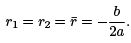We have one solution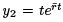. How can we ﬁnd the second solution which is linearly independent of y1?

From experience in an earlier example, we claim thatis a solution. To prove this claim, we plug it back into the equation. If r¯ is the double root, then, the characteristic equation can be written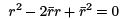which gives the equation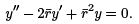We can check if y2 satisﬁes this equation. We have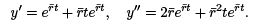Put into the equation, we get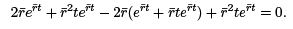Finally, we must make sure that y1, y2 are linearly independent. We compute their Wronskian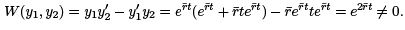We conclude now, the general solution is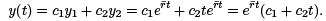Example 1. (not covered in class) Consider the equation y′′ + 4y′ + 4y = 0. We have r2 + 4r + 4 = 0, and r1 = r2 = r = −2. So one solution is y1 = e−2t . What is y2?
Method 1.
Use Wronskian and Abel’s Theorem. By Abel’s Theorem we have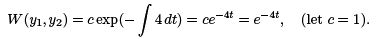By the deﬁnition of Wronskian we have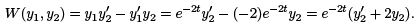They must equal to each other:Solve this for y2,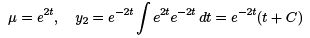Let C = 0, we get y= te−2t , and the general solution is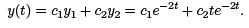Method 2. This is the textbook’s version. We guess a solution of the form y= v(t)y1 = v(t)e−2t , and try to ﬁnd the function v(t). We have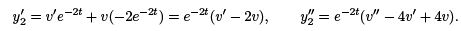Put them in the equation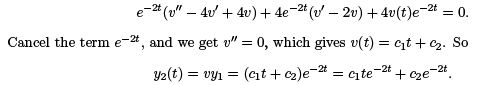Note that the term c2 e−2t is already contained in cy1 .

Therefore we can choose c= 1, c2 = 0, and get y2 = te−2t , which gives the same general solution as Method 1. We observe that this method involves more computation than Method 1.
A typical solution graph is included below: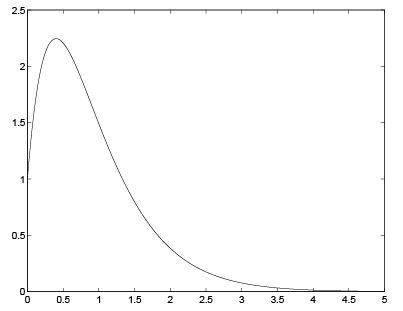We see if c2 > 0, y increases for small t. But as t grows, the exponential (decay) function dominates, and solution will go to 0 as t → ∞.

One can show that in general if one has repeated roots r1 = r2 = r, then y1 = ert and y2 = tert , and the general solution is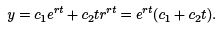Example 2. Solve the IVP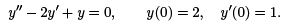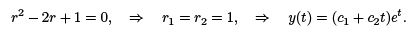The ICs give

y(0) = 2 : c+ 0 = 2, ⇒ c= 2.

y′(t) = (c1 + c2 t)et + ce, y′(0) = c1 + c2 = 1, ⇒ c2 = 1 − c1 = −1.

So the solution is y(t) = (2 − t)et .

Summary: For ay′′ + by′ + cy = 0, and ar2 + br + c = 0 has two roots r1, r2 , we have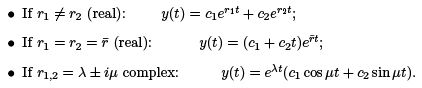On reduction of order: This method can be used to ﬁnd a second solution y2 if the ﬁrst solution yis given for a second order linear equation.

Example 3. For the equation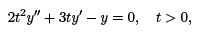given one solution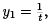ﬁnd a second linearly independent solution.

Answer.Method 1: Use Abel’s Theorem and Wronskian. By Abel’s Theorem, and choose C = 1, we have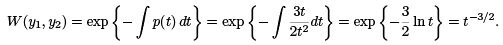By deﬁnition of the Wronskian,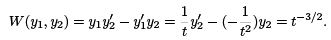Solve this for y2: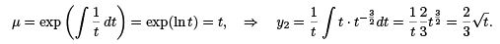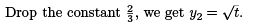Method 2. We will use Abel’s Theorem, and at the same time we will seek a solution of the form y1 = vy1.

By Abel’s Theorem, we have ( worked out in M1) W (y1, y2)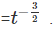. Now, seek y2 = vy1.

By the deﬁnition of the Wronskian, we have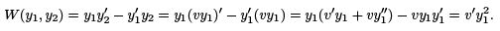Note that this is a general formula: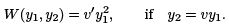Now putting y1 = 1/t, we get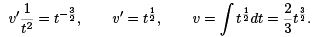Drop the constant 3/2 , we get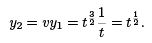We see that Method 3 is the most eﬃcient one among all three methods. We will focus on this method from now on.

Example 4. Consider the equation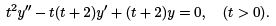Given y= t, ﬁnd the general solution.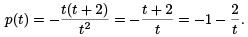Let y2 be the second solution. By Abel’s Theorem, choosing c = 1, we have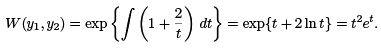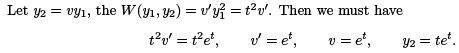(A cheap trick to double check your solution y2 would be: plug it back into the equation and see if it satisﬁes it.) The general solution is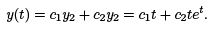We observe here that Method 2 is very eﬃcient.

Example 5. Given the equation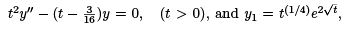ﬁnd y2

Answer.We will always use method 2. We see that p = 0. By Abel’s Theorem, setting c = 1, we have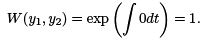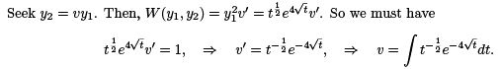So drop the constant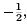, we get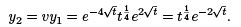The general solution is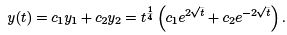Non-homogeneous equations; method of undetermined coeﬃcients

Want to solve the non-homogeneous equation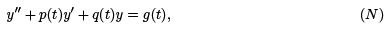Steps:

1. First solve the homogeneous equation

y′′ + p(t)y′ + q(t)y = 0,                         (H )

i.e., ﬁnd y1, y2, linearly independent of each other, and form the general solution

yH = c1 y1 + c2 y2.

2. Find a particular/speciﬁc solution Y for (N), by MUC (method of undetermined coeﬃcients);

3. The general solution for (N) is then

y = yH + Y = c1y1 + c2y2 + Y .

Find c1 , c2 by initial conditions, if given.

Key step: step 2.

Why y = yH + Y ?

A quick proof: If yH solves (H), then

y′′H + p(t)y′H + q(t)yH = 0,                 (A)

and since Y solves (N), we have

Y ′′ + p(t)Y ′ + q(t)Y = g(t),                (B )

Adding up (A) and (B), and write y = yH + Y , we get y′′ + p(t)y′ + q(t)y = g(t).

Main focus: constant coeﬃcient case, i.e.,

ay′′ + by′ + cy = g(t).

Example 1. Find the general solution for y′′ − 3y′ − 4y = 3e2t .

r2 − 3r − 4 = (r + 1)(r − 4) = 0, ⇒ r1 = −1, r2 = 4, so

yH = c1 e−t + c2 e4t.

Step 2: Find Y . We guess/seek solution of the same form as the source term Y = Ae2t , and will determine the coeﬃcient A.

Y ′ = 2Ae2t , Y ′′ = 4Ae2t .

Plug these into the equation: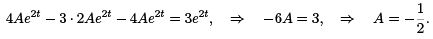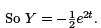Step 3. The general solution to the non-homogeneous solution is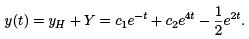Observation: The particular solution Y take the same form as the source term g(t).

But this is not always true.

Example 2. Find general solution for y′′ − 3y′ − 4y = 2e−t .

Answer.The homogeneous solution is the same as Example 1: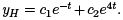For the particular solution Y , let’s ﬁrst try the same form as g, i.e., Y = Ae−t . So Y ′ = −Ae−t , Y ′′ = Ae−t . Plug them back in to the equation, we get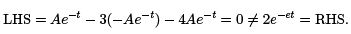So it doesn’t work. Why?

We see r1 = −1 and y1 = e−t, which means our guess Y = Ae−t is a solution to the homogeneous equation. It will never work.

Second try: Y = Ate−t . So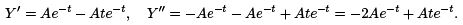Plug them in the equation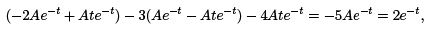we get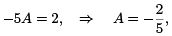so we have Y  =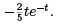Summary 1. If g(t) = aeαt , then the form of the particular solution Y depends on r1 , r2 (the roots of the characteristic equation).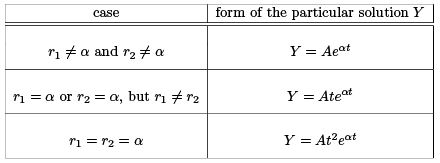Example 3. Find the general solution for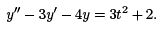Answer.The yH is the same yH =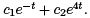Note that g(t) is a polynomial of degree 2. We will try to guess/seek a particular solution of the same form:

Y = At2 + Bt + C,       Y ′ = 2At + B,              Y ′′ = 2A

Plug back into the equation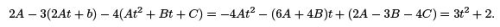Compare the coeﬃcient, we get three equations for the three coeﬃcients A, B , C :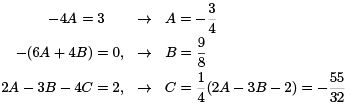So we get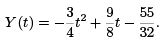But sometimes this guess won’t work.

Example 4. Find the particular solution for y′′ − 3y′ = 3t2 + 2.

Answer.We see that the form we used in the previous example Y = At2 + Bt + C won’t work because Y′′ − 3Y′ will not have the term t2 .

New try: multiply by a t. So we guess Y = t(At2 + B t + C ) = At3 + B t+ Ct. Then

Y ′ = 3At2 + 2B t + C, Y ′′ = 6At + 2B .

Plug them into the equation
(6At + 2B) − 3(3At2 + 2Bt + C ) = −9At2 + (6A − 6B)t + (2B − 3C ) = 3t2 + 2.

Compare the coeﬃcient, we get three equations for the three coeﬃcients A, B , C :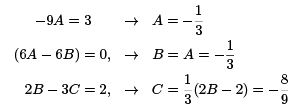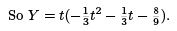Summary 2. If g(t) is a polynomial of degree n, i.e.,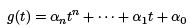the particular solution for

ay′′ + by′ + cy = g(t)

(where a = 0) depends on b, c: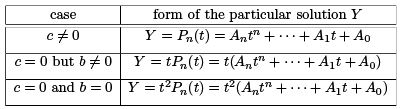Example 5. Find a particular solution for

y′′ − 3y′ − 4y = sin t.

Answer.Since g(t) = sin t, we will try the same form. Note that (sin t)′ = cos t, so we must have the cos t term as well. So the form of the particular solution is

Y = A sin t + B cos t.

Then Y ′ = A cos t − B sin t, Y ′′ = −A sin t − B cos t.

Plug back into the equation, we get (−A sin t − B cos t) − 3(A cos t − B sin t) − 4(A sin t + b cos t) = (−5A + 3B ) sin t + (−3A − 5B ) cos t = sin t.

So we must have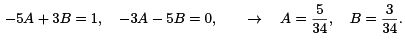So we get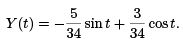We observe that: (1). If the right-hand side is g(t) = a cos t, then the same form would work; (2). More generally, if g(t) = a sin t + b sin t for some a, b, then the same form still work.

However, this form won’t work if it is a solution to the homogeneous equation.

Example 6. Find a general solution for y′′ + y = sin t.

Answer. Let’s ﬁrst ﬁnd yH . We have r2 + 1 = 0, so r1,2 = ±i, and yH = c1 cos t + c2 sin t.

For the particular solution Y : We see that the form Y = A sin t + B cos t won’t work because it solves the homogeneous equation.

Our new guess: multiply it by t, so

Y (t) = t(A sin t + B cos t).

Then

Y ′ = (A sin t + B cos t) + t(A cos t + B sin t), Y ′′ = (−2B − At) sin t + (2A − B t) cos t.

Plug into the equation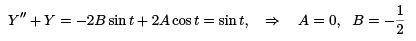So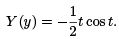The general solution is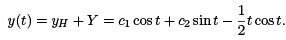Summary 3. If g(t) = a sin αt + b cos αt, the form of the particular solution depends on the roots r1 , r2.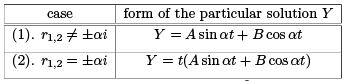Note that case (2) occurs when the equation is y′′ + α2 y = a sin αt + b cos αt.

We now have discovered some general rules to obtain the form of the particular solution for the non-homogeneous equation ay′′ + by′ + cy = g(t).

• Rule (1). Usually, Y take the same form as g(t);
• Rule (2). Except, if the form of g(t) provides a solution to the homogeneous equation.

Then, one can multiply it by t.

• Rule (3). If the resulting form in Rule (2) is still a solution to the homogeneous equation, then, multiply it by another t.

Next we study a couple of more complicated forms of g.

Example 7. Find a particular solution for

y′′ − 3y′ − 4y = te.

Answer. We see that g = P1 (t)eat , where P1 is a polynomial of degree

1. Also we see r= −1, r= 4, so r1 = a and r2 = a. For a particular solution we will try the same form as g, i.e., Y = (At + B )et. So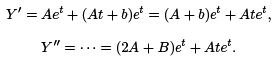Plug them into the equation,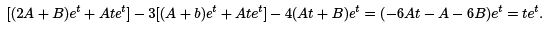We must have −6At − A − 6B = t, i.e.,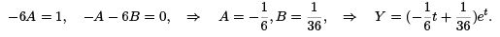However, if the form of g is a solution to the homogeneous equation, it won’t work for a particular solution. We must multiply it by t in that case.

Example 8. Find a particular solution of

y′′ − 3y′ − 4y = te−t .

Answer.Since a = −1 = r1, so the form we used in Example 7 won’t work here. (Can you intuitively explain why?)

Try a new form now Y = t(At + B )e−t = (At+ B t)e−t.
Then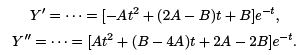Plug into the equation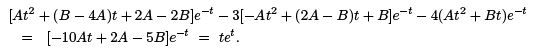So we must have −10At + 2A − 5B = t, which means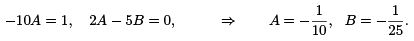Then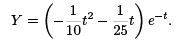Summary 4. If g(t) = Pn (t)eat where Pn(t) = αntn + · · · + α1 t + α0 is a polynomial of degree n, then the form of a particular solution depends on the roots r1, r2 .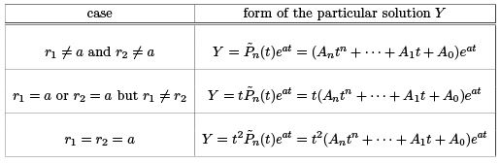Other cases of g are treated in a similar way: Check if the form of g is a solution to the homogeneous equation. If not, then use it as the form of a particular solution. If yes, then multiply it by t or t2 .

We summarize a few cases below.

Summary 5. If g(t) = eαt (a cos β t + b sin β t), and r1, r2 are the roots of the characteristic equation. Then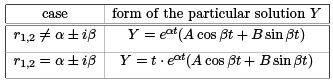Summary 6. If g(t) = Pn(t)eαt (a cos β t + b sin β t) where Pn (t) is a polynomial of degree n, and r1, r2 are the roots of the characteristic equation. Then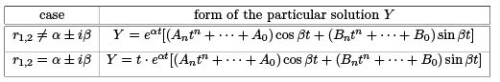More terms in the source. If the source g(t) has several terms, we treat each separately and add up later. Let g(t) = g1 (t) + g2 (t) + · · · gn (t), then, ﬁnd a particular solution Yfor each gi(t) term as if it were the only term in g, then Y = Y1 + Y2 + · · · Yn . This claim follows from the principle of superposition. (Can you provide a brief proof ?)

In the examples below, we want to write the form of a particular solution.

Example 9. y′′ − 3y′ − 4y = sin 4t + 2e4t + e5t − t.
Since r= −1, r2 = 2, we treat each term in g separately and the add up: Y (t) = A sin 4t + B cos 4t + C te4t + De5t + (E t + F ).

Example 10. y′′ + 16y = sin 4t + cos t − 4 cos 4t + 4.
.The char equation is r2 + 16 = 0, with roots r1,2 = ±4i, and yH = csin 4t + c2 cos 4t.

We also note that the terms sin 4t and −4 cos 4t are of the same type, and we must multiply it by t. So
Y = t(A sin 4t + B cos 4t) + (C cos t + D sin t) + E .

Example 11. y′′ − 2y′ + 2y = et cos t + 8et sin 2t + te−t + 4e−t + t2 − 3.
The char equation is r2 − 2r + 2 = 0 with roots r1,2 = 1 ± i. Then, for the term et cos t we must multiply by t.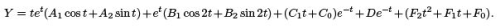159 docs

,

,

,

,

,

,

,

,

,

,

,

,

,

,

,

,

,

,

,

,

,

,

,

,

;